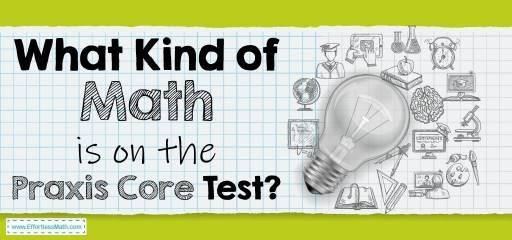# What Kind of Math Is on the Praxis Core Test?The Praxis Core is a standardized test that assesses students’ academic ability for admissions in teacher training programs in the United States. The test is administered by the Educational Testing Service (ETS). Many government licensing agencies, as well as colleges and universities, require certification of the Praxis Core test.

The Praxis Core test covers three topics: Math, Reading, Writing.

The math part of the Praxis Core test is 56 questions, some of which are multiple-choice and the rest are numeric entry questions. Test takers have 90 minutes to answer the Praxis Core Math test questions.

Test takers are not allowed to bring their calculators but are provided with an on-screen calculator.

Praxis Core Mathematics covers the following topics:

• Number and Quantity
• Algebra and Functions
• Geometry
• Data Interpretation, Statistics, and Probability

## Number and Quantity

In this section, you should be familiar with concepts such as proportions, ratios, addition, subtraction, multiplication, division, integers, radicals, and solving word problems. You must also have a thorough understanding of the order among fractions. You need to know how to represent a number in more than one way and what Properties of whole numbers are.

## Algebra and Functions

The section on algebra and functions includes topics such as operation properties, linear equations, functions, graphing, and modeling. In other words, in this section, you need to master the manipulation of expressions and equations, solving linear and quadratic equations, solving problems of algebraic words.

## Geometry

Geometrical figures, theorem circles, angles, area, volume, and geometric concepts are some of the items included in the geometry section of the Praxis Core Math test. To get the desired score in this section, you must be completely familiar with the characteristics of geometric shapes, similarities, and angle relationships.

## Data Interpretation, Statistics, and Probability

Statistics and probabilities are an important part of the test where you need to have strong analysis and interpretation skills to succeed. Interpretation of quantitative information, understanding the relationships between data, and Determining mean, median, and mode are included in this section. Assigning a probability to an outcome is also one kind of question in this part of the test.

## FAQs:

### Is the Praxis Core Math test hard?

Praxis Core test questions are usually much easier than the SAT and ACT questions.

### How do I study for the Praxis Core Math?

How you prepare for the Praxis Core Math test is very important. You can use good prep books to prepare well for the Praxis Core Math test. You can also use online practice tests for further practice after studying the Praxis Core exam courses.

### How many times can you take the Praxis test?

You can take the Praxis Core test once every 21 days.

Looking for the best resource to help you succeed on the Praxis Core Math test?

## The Best Books to Ace the Praxis Core Math Test

### What people say about "What Kind of Math Is on the Praxis Core Test? - Effortless Math: We Help Students Learn to LOVE Mathematics"?

No one replied yet.

X
52% OFF

Limited time only!

Save Over 52%

SAVE \$40

It was \$76.99 now it is \$36.99